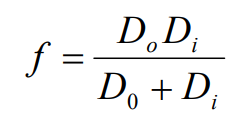Subject:
Mathematics

Topics: AlgebraExpressions and Equations
Common Core State Standard: A-CED.2, A-CED.4,
Knowledge and Skills:

· Can evaluate expressions by substituting values for variables
· Can simplify expressions using correct order of operations
· Can do basic operations on both sides of an equation in such a way as to preserve the equality

Lesson:

### Objects and Images

The focal length of a lens (f) is related to the distance from the lens to the object (Do) and the distance from the lens to the image that is created (Di) by this equation:1. Determine the focal length of each lens:

 lens Do (inches) Di (inches) f #1 25 3 #2 30 3.5 #3 100 2.75 #4 50 3.25

2. Find the missing value in each case:

 lens Do (inches) Di (inches) f #5 ? 2.25 2.09 #6 40 ? 2.57 #7 10 ? 2.45 #8 ? 2 1.94 #9 ? 2 1.96 #10 10 ? 3.00 #10 25 ? 3.00 #10 50 ? 3.00 #10 100 ? 3.00 #10 200 ? 3.00 #10 500 ? 3.00 #10 1000 ? 3.00 #10 2000 ? 3.00

For lens #10, what happens to the value of Di as Do gets very large? (Compare Di to the value of f.)